## 程序员最近都爱上了这个网站  程序员们快来瞅瞅吧！  it98k网:it98k.com

出租广告位,需要合作请联系站长

+关注

## 校招题目：用计算机生成迷宫

### 校招题目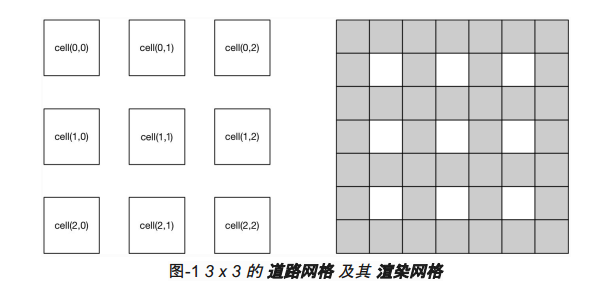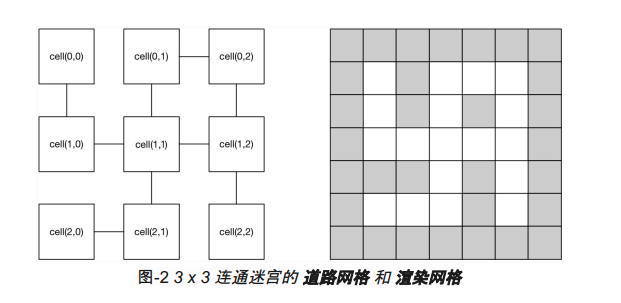● 每一个 ​cell​ 只能够直接与相邻正南、正北、正东、正西的 ​cell​ 连通。不能够和其他的 ​cell​ 连通。
● 两个 ​cell​ 之间的连通一定是双向的。即 ​cell(0,0) ​和 ​cell(1,0)​ 连通等价于 ​cell(1,0)​ 和cell(0,0)​ 的连通。

，仅做演示，实际实现时请应用实际语言的编程风格）
Maze maze = MazeFactory.Create(command);
String mazeText = maze.Render();

● 第一行是迷宫 ​道路网格​ 的尺寸。例如 3 x 3 的迷宫为 ​3 3​，而 5 x 4 的迷宫为 ​5 4​（5 行 4 列）​。
● 第二行是迷宫 ​道路网格​ 的连通性定义。如果 ​cell(0,1) ​和​ cell(0,2) ​是连通的，则表示为：
0,1 0,2​，多个连通以分号 ​; ​隔开。

3 3
0,1 0,2;0,0 1,0;0,1 1,1;0,2 1,2;1,0 1,1;1,1 1,2;1,1 2,1;1,2 2,2;2,0 2,1

[W] [W] [W] [W] [W] [W] [W]
[W] [R] [W] [R] [R] [R] [W]
[W] [R] [W] [R] [W] [R] [W]
[W] [R] [R] [R] [R] [R] [W]
[W] [W] [W] [R] [W] [R] [W]
[W] [R] [R] [R] [W] [R] [W]
[W] [W] [W] [W] [W] [W] [W]

#### 使用语言：python

``````import re

class FormatError(Exception):
pass

try:
while(1):
m = int(input("\nm = "))
n = int(input("n = "))
N = [['[W]']*(n*2+1) for i in range(m*2+1)]

for x in range(m*2+1):
for y in range(n*2+1):
if x%2 == 1 and y%2 == 1:
N[x][y] = "[R]"

for i in range(m*2+1):
print("\n")
for j in range(n*2+1):
print(N[i][j],end=" ")
print("\n\ninput way to go: ")
line = input()
linestr = line.split(";")
for i in range(0, len(linestr)):
r = re.split(r"[\s+\,]",linestr[i])
for j in range(0, 4):
if(r!=r and r!=r):
raise FormatError("Maze format error.")
else:
N[int(r)+int(r)+1][int(r)+int(r)+1] = "[R]"

for i in range(m*2+1):
print("\n")
for j in range(n*2+1):
print(N[i][j],end=" ")
except FormatError as e:
print(e)
except NameError:
print("Invalid number format​.")
except ValueError:
print("Incorrect command format​.​​")
except IndexError:
print("Number out of range​.")
except KeyboardInterrupt:
print("Programme quit.")
```123456789101112131415161718192021222324252627282930313233343536373839404142434445```

1、首先运行程序，输入迷宫的高（m）和宽（n）: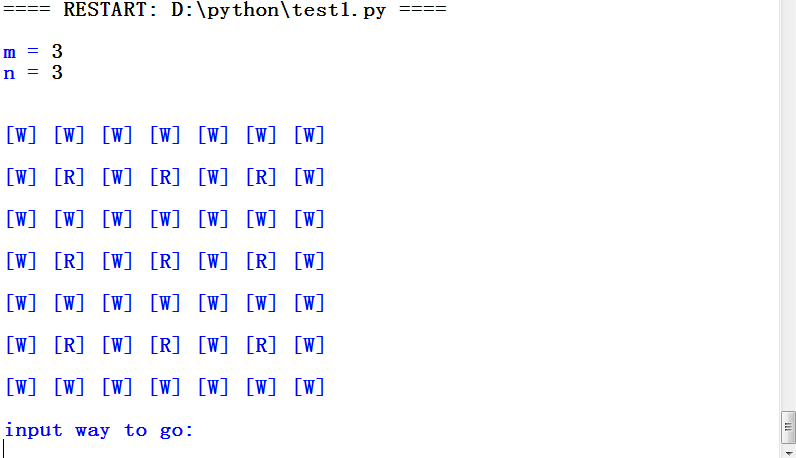2、接着选择去哪个方向的道路，它会生成如下的图：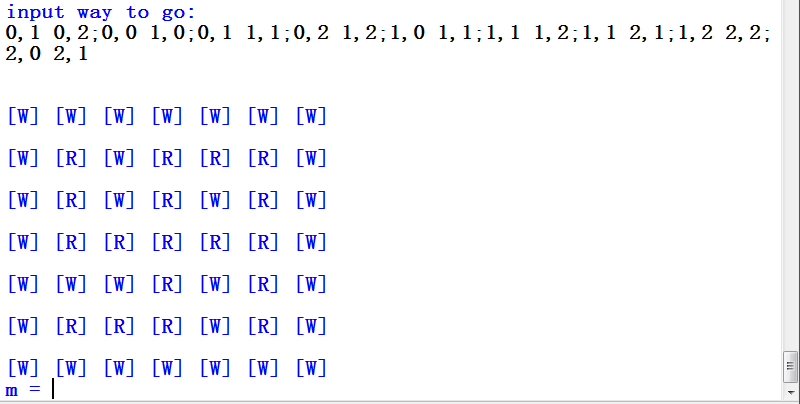3、接着你又可以构造自己的迷宫，但是当选择的路无法连通时，会出现如下问题：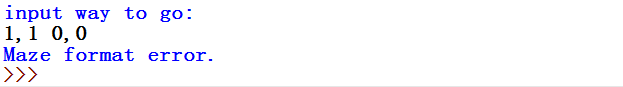3 0

pdf(new) 更多>